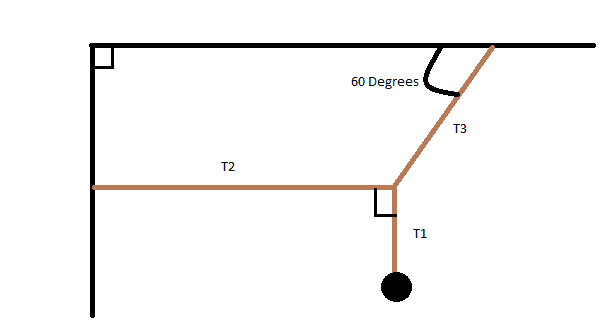# Equilibrium Question With Ropes and Weight

## Homework StatementClick on photo to enlarge

The weight at the end of the rope is 1000 N. Find tensions in T1, T2, and T3.

## Homework Equations

Law of Cosine: a^2+b^2-2ab(cosC)=c^2
SOH-CAH-TOA

## The Attempt at a Solution

T1 has to be 1000N. I figured T2 and T3 have to add up to the weight of 1000 N. I ended up added the vectors head to tail and found T2 to be 577 N and T3 would have to be 1000-577=423 N since both ropes have to add up to 1000 N to be in equilibrium. I honestly do not have a clue how to do the problem (it was on a test today and it's really bugging me what the answer is or how to even achieve the answer)